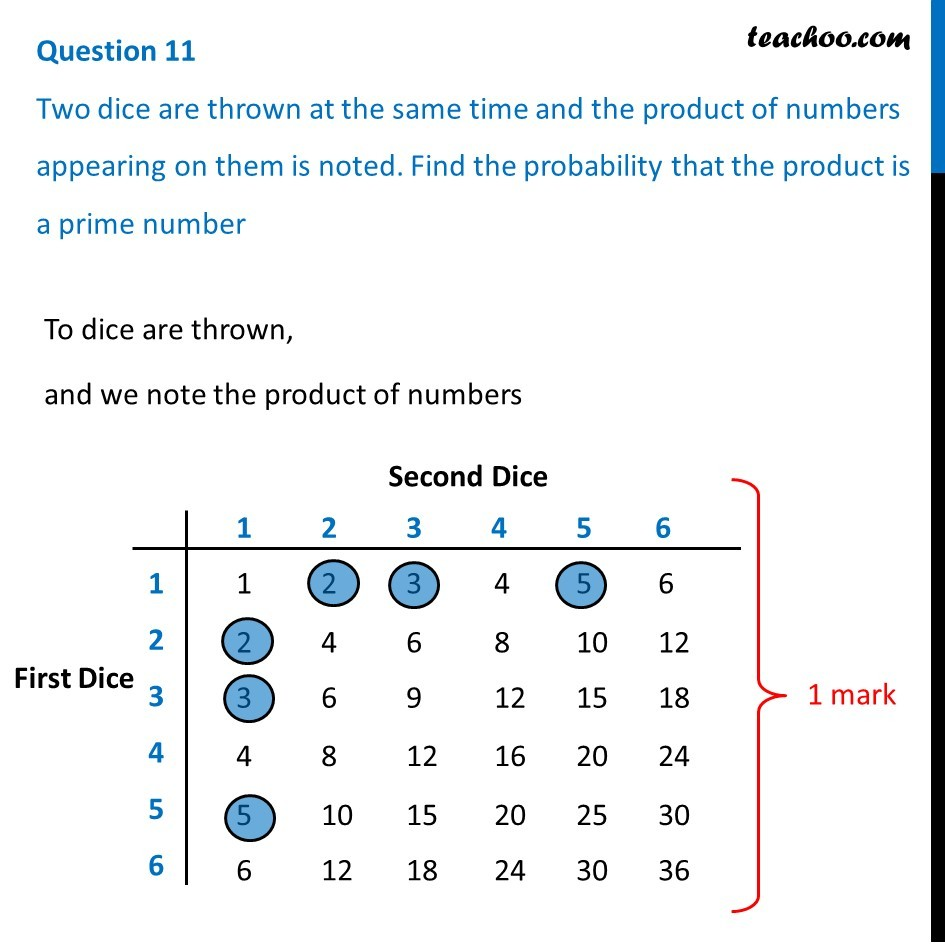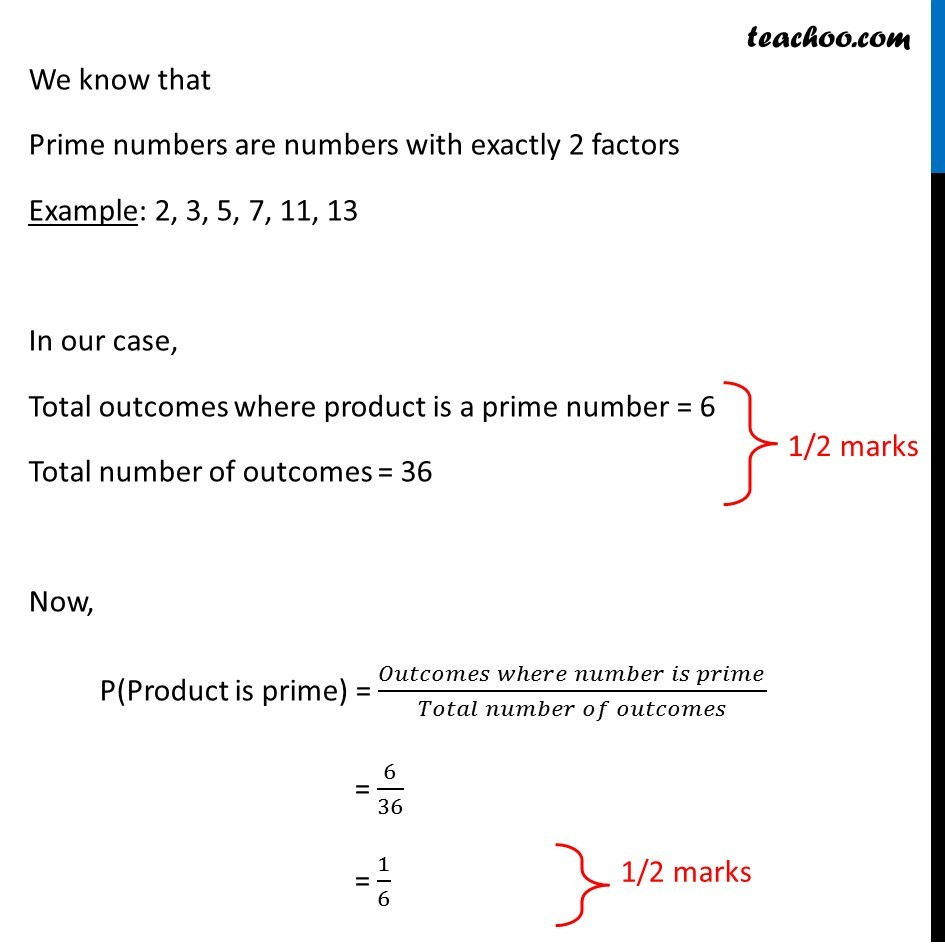CBSE Class 10 Sample Paper for 2019 Boards

Class 10
Solutions of Sample Papers for Class 10 Boards

Question 11

Two dice are thrown at the same time and the product of numbers appearing on them is noted. Find the probability that the product is a prime numberLearn in your speed, with individual attention - Teachoo Maths 1-on-1 Class

### Transcript

Question 11 Two dice are thrown at the same time and the product of numbers appearing on them is noted. Find the probability that the product is a prime number To dice are thrown, and we note the product of numbers We know that Prime numbers are numbers with exactly 2 factors Example: 2, 3, 5, 7, 11, 13 In our case, Total outcomes where product is a prime number = 6 Total number of outcomes = 36 1/2 marks Now, P(Product is prime) = (𝑂𝑢𝑡𝑐𝑜𝑚𝑒𝑠 𝑤ℎ𝑒𝑟𝑒 𝑛𝑢𝑚𝑏𝑒𝑟 𝑖𝑠 𝑝𝑟𝑖𝑚𝑒)/(𝑇𝑜𝑡𝑎𝑙 𝑛𝑢𝑚𝑏𝑒𝑟 𝑜𝑓 𝑜𝑢𝑡𝑐𝑜𝑚𝑒𝑠) = 6/36 = 1/6 1/2 marks